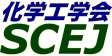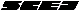## \$B9V1i%W%m%0%i%`!J2q>l!&F|DxJL!K(B

### N\$B2q>l(B

\$B:G=*99?7F|;~!'(B2012-08-20 15:02:17

\$BBh(B1\$BF|(B
\$B9V1i(B
\$B;~9o(B
\$B9V1i(B
\$BHV9f(B
\$B9V1iBjL\!?H/I=\$B%-!<%o!<%I(B\$BJ,N`(B
\$BHV9f(B
\$B\$BHV9f(B
\$B%7%s%]%8%&%`(B \$B!cG.J*
(9:00\$B!A(B10:00)\$B!!(B(\$B:BD9(B \$BLZ86(B \$B?-0l(B)
9:00\$B!A(B 9:20N101\$BJ#9g1UE)\$N@8@.%7%_%e%l!<%7%g%s(B
(\$B:k6LBg1!M}9)(B) \$B!{(B(\$B@5(B)\$BK\4V(B \$B=S;J(B \$B!&(B (\$B3X(B)\$B?98}(B \$B8xB@(B \$B!&(B \$B%-%`(B \$B%F%=%s(B \$B!&(B (\$B@5(B)\$B8E4W(B \$BFsO:(B
Compound Drop
Dual Nozzle
Numerical Simulation
S-27122
9:20\$B!A(B 9:40N102\$B2=3XH?1~\$K\$h\$k3&LLD%NO8:>/\$rH<\$&(BViscous Fingering\$B\$K4X\$9\$k
(\$BElG@9)Bg1!(B) \$B!{(B(\$B3X(B)\$BF#B<(B \$B>-@.(B \$B!&(B (\$B@5(B)\$BD9DE(B \$BM:0lO:(B \$B!&(B (\$BL>9)Bg1!(B) (\$B@5(B)\$BB?ED(B \$BK-(B
Viscous Fingering
\$B@PL}(B
\$B%"%k%+%j8yK!(B
S-27420
(\$B6eBg1!9)(B) \$B!{(B(\$B@5(B)\$B>>7((B \$BMN2p(B \$B!&(B (\$B6eBg9)(B) \$B;e2l(B \$BN<2p(B \$B!&(B (\$B6eBg1!9)(B) (\$B@5(B)\$BJw85(B \$B2m
droplet
surface tension
porous material
S-27530
(10:00\$B!A(B11:00)\$B!!(B(\$B:BD9(B \$B4dED(B \$B=\$0l(B)
10:00\$B!A(B 10:20N1042\$B@.J,J#9g:`NA\$N9=B\$:GE,2=\$K\$h\$k%H%]%m%8!<@_7W(B
(\$B:kBg1!M}9)(B) \$B!{(B(\$B@5(B)\$B8E4W(B \$BFsO:(B \$B!&(B \$B8E4W(B \$B=c0l(B \$B!&(B (\$B@5(B)\$BK\4V(B \$B=S;J(B
Composite materials
Structural optimization
Topology optimization
S-2754
10:20\$B!A(B 10:40N105\$B%]%j%^!<(B/\$BM-5!MOG^7O\$N\$;\$sCGG4EY\$NG;EY\$*\$h\$S05NO0MB8@-(B
(\$B9-Bg1!9)(B) \$B!{(B(\$B@5(B)\$BLZ86(B \$B?-0l(B \$B!&(B (\$B9-Bg9)(B) \$B@6?e(B \$BN4@5(B \$B!&(B \$BD%(B \$B?7MN(B \$B!&(B (\$B9-Bg1!9)(B) (\$B@5(B)\$B=ULZ(B \$B>-;J(B \$B!&(B (\$B@5(B)\$BBlV:(B \$BHK
branched polymer melts
shear viscosity
high pressure
S-27625
10:40\$B!A(B 11:00N106\$B9bJ,;R?eMO1U\$N?-D9G4EYA}2C\$,MpN.M^@)\$K5Z\$\\$91F6A\$N2rL@(B
(\$B?@8MBg<+(B) \$B!{(B(\$B@5(B)\$BF|=P4V(B \$B\$k\$j(B \$B!&(B (\$B?@8MBg1!9)(B) (\$B@5(B)\$BNkLZ(B \$BMN(B \$B!&(B (\$B@5(B)\$B8VED(B \$B1YG7(B \$B!&(B (\$B?@8MBg(B) \$B5W>>(B \$B;K2:(B \$B!&(B \$BGrLZ(B \$BG=?N(B \$B!&(B (\$B;37ABg1!M}9)(B) \$B8E@n(B \$B1Q8w(B
\$BMpN.M^@)(B
\$B9bJ,;R(B
\$B?-D9G4EY(B
S-27253
(11:00\$B!A(B12:00)\$B!!(B(\$B:BD9(B \$BK\4V(B \$B=S;J(B)
11:00\$B!A(B 11:20N107\$B29EY:9\$HG;EY:9\$K5/0x\$9\$k%^%i%s%4%KBPN.\$NHsDj>o@-\$N@)8f\$K4X\$9\$k?tCM2r@O(B
(\$BN0Bg9)(B) \$B!{(B(\$B@5(B)\$B?e8}(B \$B>0(B \$B!&(B (\$BN0Bg1!M}9)(B) \$BDiED(B \$BM*5*(B \$B!&(B (\$BN0Bg9)(B) \$B574V(B \$B8g(B \$B!&(B (\$B:eBg1!4p9)(B) (\$B@5(B)\$B9bLZ(B \$BMNJ?(B \$B!&(B (\$B@5(B)\$B2,Ln(B \$BBYB'(B
Marangoni convection
Floating zone technique
Rotation effect
S-2772
11:20\$B!A(B 11:40N108\$B1UKlFb%^%i%s%4%KBPN.\$K5Z\$\\$9%a%K%9%+%97A>u\$N1F6A\$K4X\$9\$k?tCM2r@O(B
(\$B:eBg1!4p9)(B) \$B!{(B(\$B3X(B)\$B;3K\(B \$BBnLi(B \$B!&(B (\$B@5(B)\$B9bLZ(B \$BMNJ?(B \$B!&(B (\$B@5(B)\$BH<(B \$B5.I'(B \$B!&(B (\$B@5(B)\$B2,Ln(B \$BBYB'(B \$B!&(B (\$BN0Bg9)(B) (\$B@5(B)\$B?e8}(B \$B>0(B
Thermocapillary
Meniscus
Numerical simulation
S-2782
11:40\$B!A(B 12:00N109\$BD@EBH?1~\$,0z\$-5/\$3\$9N.BNNO3XE*IT0BDj@-\$K4X\$9\$k8&5f(B
(\$BL>9)Bg(B) \$B!{(B(\$B3X(B)\$B@P0f(B \$BM\$5*(B \$B!&(B (\$BElG@9)Bg(B) (\$B@5(B)\$BD9DE(B \$BM:0lO:(B \$B!&(B (\$BL>9)Bg(B) (\$B@5(B)\$BB?ED(B \$BK-(B \$B!&(B (ULB) Anne De Wit \$B!&(B (\$BL>9)Bg(B) (\$B@5(B)\$B2CF#(B \$BDw?M(B
Precipitation
Instability
Reacting Flow
S-2788
(13:00\$B!A(B14:00)\$B!!(B(\$B:BD9(B \$BK\4V(B \$B=S;J(B)
13:00\$B!A(B 13:20N113[\$BM%=(O@J8>^(B]\$BD69bB.2sE>%Y%k%+%C%WEIAu5!\$rBP>]\$H\$7\$?EICe8zN(\$rDc2<\$5\$;\$k0x;R\$N?tCM2r@OE*8!F\$(B
(\$BElKLBg1!9)(B) \$B!{0BB<(B \$B8wB@O:(B \$B!&(B (\$B@5(B)\$Bc7F#(B \$BBYMN(B \$B!&(B \$B>1;R(B \$B@5OB(B \$B!&(B (\$B@5(B)\$B>>2<(B \$BMN2p(B \$B!&(B (\$B@5(B)\$B@DLZ(B \$B=(G7(B \$B!&(B \$B;01:(B \$BN4Mx(B \$B!&(B (\$BH,8M9)Bg(B) \$B>.3^86(B \$B?5(B \$B!&(B (\$B@5(B)\$BBg9u(B \$B@5IR(B \$B!&(B (\$B90A0BgM}9)(B) \$B>kED(B \$BG@(B \$B!&(B \$B0pB<(B \$BN4IW(B
Numerical simulation
Rotary bell-cup atomizer
Spray flow
S-27121
13:20\$B!A(B 14:00N114[\$B>7BT9V1i(B]\$BH?1~4o3+H/\$X\$NH?1~!&N.F02r@O\$NE,MQ;vNc\$N>R2p(B
(\$B@iBeED%"%I%P%s%9%H!&%=%j%e!<%7%g%s%:(B) \$B!{(B(\$B@5(B)\$BED8}(B \$BCR>-(B
Computational Fluid Dynamics
Methane Decomposition
Reaction Engineering
S-27271
(14:00\$B!A(B15:00)\$B!!(B(\$B:BD9(B \$BA0@n(B \$B=!B'(B)
14:00\$B!A(B 14:20N116\$BHDI=LL\$K\$*\$1\$k1UKlN.\$N?tCMM=B,5Z\$S8!>Z(B
(IHI) \$B!{(B(\$B@5(B)\$B2+(B \$B7r(B \$B!&(B \$B2CF#(B \$B??M};R(B \$B!&(B \$B0k(B \$BNI9T(B \$B!&(B \$B>>Ln(B \$B?-2p(B
Gas-Liquid Two-Phase Flow
Wetting Flow
CFD
S-27194
14:20\$B!A(B 14:40N117\$B%+!<%\%s%V%i%C%/H?1~O'\$NMpN.G.J*
(\$B00%+!<%\%s(B) \$B!{J!ED(B \$B6=>H(B \$B!&(B (\$B@5(B)\$B;38}(B \$BEl8c(B \$B!&(B (\$B?73cBg9)(B) \$B>>86(B \$B9,<#(B \$B!&(B \$B]/0f(B \$BFF(B \$B!&(B (\$BEENO7W;;%;%s%?!<(B) \$Bt"(B \$BH~@iBe(B \$B!&(B \$BFsB<(B \$B>;
Carbon Black
Simulation
PDF model
S-27222
14:40\$B!A(B 15:00N118\$B5\$1U3&LL\$rMxMQ\$7\$?9b<}N(%j%]%=!<%`@8@.K!\$N3+H/(B
(\$B?@8MBg1!9)(B) \$B!{(B(\$B3X(B)\$BAj86(B \$B9(I'(B \$B!&(B (\$B@5(B)\$BNkLZ(B \$BMN(B \$B!&(B (\$B@5(B)\$B>!ED(B \$BCN>0(B \$B!&(B (\$B@5(B)\$B8VED(B \$B1YG7(B \$B!&(B (\$B?@8MBg<+(B) (\$B@5(B)\$BF|=P4V(B \$B\$k\$j(B
liposome
micro-channel
S-27274
(15:00\$B!A(B16:00)\$B!!(B(\$B:BD9(B \$B>>7((B \$BMN2p(B)
15:00\$B!A(B 15:20N119\$BD62;GHH?1~4oFb\$N%Q%k%9>H
(\$BL>9)Bg(B) (\$B@5(B)\$BB?ED(B \$BK-(B \$B!&(B \$B!{(B(\$B3X(B)\$BJ?@n(B \$B3>B@O:(B \$B!&(B (\$B@5(B)\$B2CF#(B \$BDw?M(B
ultrasonic
pressure distribution
hydrophone
S-27806
15:20\$B!A(B 15:40N120\$B?eJ?N.O)Fb\$K\$*\$1\$kD>8rJ#9gBPN.G.EAC#(B
(\$B;37ABg1!M}9)(B) \$B!{(B(\$B3X(B)\$B?@D9(B \$B?? \$B!&(B (\$B@5(B)\$BLg3p(B \$B=( \$B!&(B (\$B@5(B)\$B86ED(B \$B1QFs(B \$B!&(B (\$B@5(B)\$B7*;3(B \$B2mJ8(B
Mixed convection
Heat transfer coefficient
Simulation
S-2723
15:40\$B!A(B 16:00N121\$BD>8r5\$N.Cf\$K\$*\$1\$k2CG.?eJ?Fs1_Cl<~\$j\$N43>D\$rH<\$&6/@)BPN.G.EAC#(B
(\$B;37ABg1!M}9)(B) \$B!{(B(\$B3X(B)\$B9b66(B \$B?NH~(B \$B!&(B (\$B@5(B)\$BLg3p(B \$B=( \$B!&(B (\$B@5(B)\$B86ED(B \$B1QFs(B \$B!&(B (\$B@5(B)\$B7*;3(B \$B2mJ8(B
Forced convection
Heat transfer coefficient
Cylinder Array
S-2726
(16:00\$B!A(B17:00)\$B!!(B(\$B:BD9(B \$BLg3p(B \$B=(
16:00\$B!A(B 16:20N122\$B>.7?!&9b51EY(BLED\$B?eCfEt\$K\$*\$1\$kJ|G.%7%9%F%`\$N3+H/(B
(\$B \$B!&(B \$B6aF#(B \$B0!4u;R(B \$B!&(B \$BEl@n(B \$BCR4u(B \$B!&(B (\$B@5(B)\$BE{0f(B \$B=SM:(B
flat heat pipe
high-intensity LED light system
natural cooling
S-27845
16:20\$B!A(B 16:40N123GPGPU\$B\$K\$h\$k(BOpenLBMflow\$B\$NJBNs7W;;(B
(\$B@EBg9)(B) \$B!{(B(\$B3X(B)\$B2#0f(B \$B8g(B \$B!&(B (\$B3X(B)\$BH<(B \$BOB \$B!&(B \$B;{ED(B \$B=(J8(B \$B!&(B (\$B@5(B)\$B@%Ln(B \$BCi0&(B
GPGPU
LBM
Numerical Simulation
S-27843
16:40\$B!A(B 17:00N124GPGPU\$B\$H(BMPS\$BK!\$K\$h\$kG4CF@-N.BN\$N2r@O(B
(\$B@EBg9)(B) \$B!{(B(\$B3X(B)\$B;3K\(B \$B9@;J(B \$B!&(B (\$B3X(B)\$BH<(B \$BOB \$B!&(B \$B;{ED(B \$B=(J8(B \$B!&(B (\$B@5(B)\$B@%Ln(B \$BCi0&(B
GPGPU
MPS
Numerical Simulation
S-27863

\$BBh(B2\$BF|(B
\$B9V1i(B
\$B;~9o(B
\$B9V1i(B
\$BHV9f(B
\$B9V1iBjL\!?H/I=\$B%-!<%o!<%I(B\$BJ,N`(B
\$BHV9f(B
\$B\$BHV9f(B
\$B%7%s%]%8%&%`(B \$B!c%W%i%:%^%W%m%;%C%7%s%0\$K\$h\$k2=3X9)3X\$N?7E83+!d(B
(9:00\$B!A(B10:00)\$B!!(B(\$B:BD9(B \$B9b66(B \$B7{;J(B)
9:00\$B!A(B 9:20N201SPCP\$BH?1~4o\$rMQ\$\$\$?(BVOC\$BJ,2r\$KBP\$9\$k9=@.EE6K>r7o\$N1F6A(B
(\$BAO2ABg9)(B) \$B!{(B(\$B3X(B)\$B@V0f(B \$B>H5W(B \$B!&(B (\$B@5(B)\$BEOIt(B \$BAO;N(B \$B!&(B (\$B@5(B)\$B0fED(B \$B=\0l(B \$B!&(B (\$B@5(B)\$B>>;3(B \$BC#(B \$B!&(B (\$B@5(B)\$B;3K\(B \$B1QIW(B
Surface discharge
Ceramic filter
Discharge electrode
S-4715
9:20\$B!A(B 9:40N202SPCP\$BH?1~4o\$K\$h\$k4xH/@-M-5!2=9gJ*\$N=hM}\$H\$=\$NAuCVFC@-(B
(\$BAO2ABg9)(B) \$B!{(B(\$B3X(B)\$B6bA}(B \$B51I'(B \$B!&(B (\$B@5(B)\$BEOIt(B \$BAO;N(B \$B!&(B (\$B@5(B)\$B0fED(B \$B=\0l(B \$B!&(B (\$B@5(B)\$B>>;3(B \$BC#(B \$B!&(B (\$B@5(B)\$B;3K\(B \$B1QIW(B
Surface discharge
Ceramic filter
Discharge states
S-4753
9:40\$B!A(B 10:00N203\$BBg5\$05%W%i%:%^%8%'%C%H\$H1UAj\$H\$N@\?(H?1~!=(BWeissler\$BK!\$K\$h\$k;@AG
(\$B@iMU9)Bg9)(B) \$B!{(B(\$B@5(B)\$BJ!2,(B \$BBgJe(B \$B!&(B \$B0KF#(B \$BIK(B \$B!&(B (\$B%j%P!<%Y%k(B) \$B>b%v9>(B \$B@5L&(B \$B!&(B (\$B@iMU9)Bg9)(B) (\$B@5(B)\$BHx>e(B \$B70(B
atmospheric plasma
oxidation
liquid phase
S-4138
(10:00\$B!A(B11:20)\$B!!(B(\$B:BD9(B \$BK\4V(B \$B=S;J(B)
10:00\$B!A(B 10:20N204\$B1UBN3&LL\$G\$N%W%i%:%^2=3XH?1~\$NJ,8wB,Dj\$*\$h\$S%P%\$%*%^%9=hM}\$X\$N1~MQ(B
(\$B6bBtBgM}9)8&5f0h(B) \$B!{(B(\$B3X(B)\$B;3@%(B \$BN<(B \$B!&(B (\$B3X(B)\$B9u_7(B \$BCNN\$(B \$B!&(B \$B2C2l(B \$B=S \$B!&(B \$B@PEg(B \$BC#IW(B \$B!&(B (\$B@5(B)\$B9b66(B \$B7{;J(B
plasma
biomass
S-4573
10:20\$B!A(B 10:40N205\$BB?Aj8rN.%"!<%/\$N9b29>l\$N@)8f\$K\$h\$k%\$%s%U%i%\$%H%,%i%9MOM;\$N9b8zN(2=(B
(\$BEl9)Bg1!AmM}9)(B) \$B!{(B(\$B@5(B)\$BEDCf(B \$B3X(B \$B!&(B \$BN-(B \$B2mhL(B \$B!&(B \$BCS>l(B \$BM' \$B!&(B \$BVC(B \$B=( \$B!&(B (\$B@5(B)\$BEOJU(B \$BN49T(B
Thermal plasma
Multi-phase arc
In-flight melting
S-4673
10:40\$B!A(B 11:00N206\$B%W%i%:%^;Y1gG3>F2<\$K\$*\$1\$kJ,8w4o\$rMQ\$\$\$?H?1~29EYB,Dj(B
(\$B0&CNEE5!(B) \$B!{EDCf(B \$BNI(B \$B!&(B (\$B6eBg9)(B) (\$B@5(B)\$B;3K\(B \$B9d(B \$B!&(B (\$B4tIlBg9)(B) (\$B@5(B)\$BHDC+(B \$B5A5*(B
Non-thermal Plasma
Plasma-assisted Combustion
temperature measurement
S-4127
11:00\$B!A(B 11:20N207\$B%W%i%:%^%W%m%;%9\$K\$*\$1\$k%,%9N.\$NLr3d\$K\$D\$\$\$F(B
(\$B7WB,%(%s%8%K%"%j%s%0%7%9%F%`(B) \$B!{%H%s(B \$B%j%A%e(B
Plasma processing
Fluid flow
Coupled simulation
S-4655
(11:20\$B!A(B12:00)\$B!!(B(\$B;J2q(B \$BHx>e(B \$B70(B)
11:20\$B!A(B 12:00N208[\$BE8K>9V1i(B] \$B?7\$7\$\$Bg5\$05%W%i%:%^AuCV\$N3+H/\$HI=LL=hM}\$*\$h\$S0eNEJ,Ln\$X\$N1~MQ(B
(\$BEl9)Bg(B) \$B!{2-Ln(B \$B98=S(B \$B!&(B \$BBg2<(B \$B5.Li(B \$B!&(B \$B9b>>(B \$BMx42(B \$B!&(B \$BCfEg(B \$B>05*(B \$B!&(B \$B5\86(B \$B=(0l(B
Atmospheric plasma
Surface treatment
Plasma medicine
S-4172
(13:00\$B!A(B13:40)\$B!!(B(\$B;J2q(B \$BEOJU(B \$BN49T(B)
13:00\$B!A(B 13:40N213[\$BE8K>9V1i(B] \$BBg5\$05%W%i%:%^=hM}\$K\$h\$k9bJ,;RI=LL2~
(\$B=UF|EE5!(B) \$B!{EDB<(B \$BK-(B \$B!&(B \$BBgDm(B \$B\F0l(B
Atmospheric plasma
Surface modification
High molecular compound
S-4980
(13:40\$B!A(B14:20)\$B!!(B(\$B:BD9(B \$BHDC+(B \$B5A5*(B)
13:40\$B!A(B 14:00N215\$B%,%9F3F~?eCf%"!<%/J|EE\$K\$h\$k6/<'@-%J%NN3;RJ,;6%+!<%\%s%J%N%[!<%s\$N9g@.(B
(\$B5~Bg1!9)(B) \$B!{(B(\$B3X(B)\$BJ?4V(B \$BBg2p(B \$B!&(B (\$B@5(B)\$B:4Ln(B \$B5*>4(B \$B!&(B (\$B@5(B)\$BEDLg(B \$BH%(B
nanoparticle
carbon nanohorn
arc discharge
S-4297
14:00\$B!A(B 14:20N216\$B%"!<%/J|EE\$K\$h\$k(BPd-Ni\$B9g6bJ,;6%+!<%\%s%J%N%[!<%s\$N9g@.\$*\$h\$S\$=\$N?eAG5[B"FC@-(B
(\$B5~Bg1!9)(B) \$B!{(B(\$B@5(B)\$B:4Ln(B \$B5*>4(B \$B!&(B \$BC+8}(B \$B9,=u(B \$B!&(B (\$B@5(B)\$BEDLg(B \$BH%(B
arc discharge
carbon nanohorn
hydrogen
S-4897
(14:20\$B!A(B15:20)\$B!!(B(\$B:BD9(B \$B:4Ln(B \$B5*>4(B)
14:20\$B!A(B 14:40N217\$BC:AG
(\$B4tIlBg9)(B) \$B!{(B(\$B3X(B)\$B86(B \$B7 \$B!&(B (\$B@5(B)\$BHDC+(B \$B5A5*(B
microwave plasma
tar
carbon nano-material
S-4392
14:40\$B!A(B 15:00N218\$B9b<~GHG.%W%i%:%^\$K\$h\$kB?%[%&2=J*%J%NN3;R\$N9g@.(B
(\$BEl9)BgAmM}9)(B) \$B!{(B(\$B@5(B)\$BEOJU(B \$BN49T(B \$B!&(B \$B>>Hx(B \$B \$B!&(B \$BVC(B \$B=(
thermal plasma
RF plasma
boride nanoparticle
S-4970
15:00\$B!A(B 15:20N219\$B9b<~GHG.%W%i%:%^\$rMxMQ\$7\$?L5?eOG@14V?P\$NLO5
(\$BEl9)BgAmM}9)(B) \$B!{(B(\$B@5(B)\$BEOJU(B \$BN49T(B \$B!&(B \$BH,LZ2<(B \$B>-;K(B \$B!&(B \$BVC(B \$B=( \$B!&(B (\$B5~BgM}(B) \$B>>Ln(B \$B=_Li(B \$B!&(B \$BEZ!G;3(B \$BL@(B
thermal plasma
RF plasma
nanoparticle
S-4206

\$B9V1i%W%m%0%i%`(B
\$B2=3X9)3X2q(B \$BBh(B44\$B2s=)5(Bg2q(B(C) 2012 \$B8x1W
Most recent update: 2012-08-20 15:02:17
For more information contact \$B2=3X9)3X2qElKL;YIt(B \$BBh(B44\$B2s=)5(Bg2q(B \$BLd\$\$9g\$;78(B
E-mail: inquiry-44fwww3.scej.org
This page was generated byeasp 2.28; proghtml 2.28c (C)1999-2011 kawase